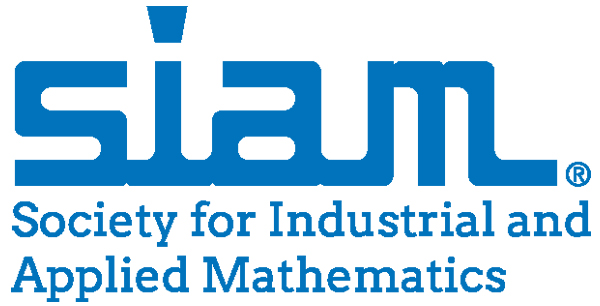## A fast non regularized numerical algorithm for solving bilevel denoising problems

We study bilevel optimization problems restricted to variational inequalities of the second kind with application to image denoising tasks. Several learning problems in image processing can be formulated in the context of a bilevel optimization problem. In such problems the lower level corresponds to a variational formulation for a specific imaging task (denoising, inpainting, segmentation, etc.) and the higher level problem uses the solution provided by the lower level to compute a quality measure using a training dataset. Even though previous work has been proposed by using a smoothed version of the lower level problem in order to characterize an optimality condition, we will focus our efforts on a non-smoothed version of the non-differentiable terms. We will find optimality conditions of the bilevel problem by exploting the directional differentiability of the solution operator of the lower level problem and propose a numeric scheme based on a Trust Region algorithm in order to find candidate solutions.## A fast non regularized numerical algorithm for solving bilevel denoising problems

We study bilevel optimization problems restricted to variational inequalities of the second kind with application to image denoising tasks. Several learning problems in image processing can be formulated in the context of a bilevel optimization problem. In such problems the lower level corresponds to a variational formulation for a specific imaging task (denoising, inpainting, segmentation, etc.) and the higher level problem uses the solution provided by the lower level to compute a quality measure using a training dataset. Even though previous work has been proposed by using a smoothed version of the lower level problem in order to characterize an optimality condition, we will focus our efforts on a non-smoothed version of the non-differentiable terms. We will find optimality conditions of the bilevel problem by exploting the directional differentiability of the solution operator of the lower level problem and propose a numeric scheme based on a Trust Region algorithm in order to find candidate solutions.## Procesamiento Matemático de Imágenes: de la teoría a la práctica

Haremos una breve revisión a los métodos variacionales clásicos utilizados para resolver problemas de procesamiento de imágenes.## Solving Bilevel Optimization Problems in Image Processing via Inexact Trust Region Methods

In this work we’ll look at the numerical solution of the problem of parameter learning in Total Variation (TV) image denoising models. The parameter learning will be based on training samples used within a PDE optimization problem. We will propose an Inexact Trust Region Algorithm and compare its performance with other numerical methods such as Semi-smooth Newton and Quasi-Newton Methods.## Solving Bilevel Optimization Problems in Image Processing via Inexact Trust Region Methods

In this work we’ll look at the numerical solution of the problem of parameter learning in Total Variation (TV) image denoising models. The parameter learning will be based on training samples used within a PDE optimization problem. We will propose an Inexact Trust Region Algorithm and compare its performance with other numerical methods such as Semi-smooth Newton and Quasi-Newton Methods.## SVM for Object Detection

Object detection is considered to be one of the most challenging computer vision tasks, it consists in finding instances of generic objects in images and video. The object detection process consist of three basic components: object search, feature extraction and classification algorithm. One of the most popular classication techniques for the object detection task is Support Vector Machine (SVM). We will make a short review of the SVM optimization problem and Kernel Functions. Even though this classifier can make use of kernel functions to discriminate data more accurately, most of the existing applications make use of linear kernels. We argue that the use of different kernels will improve the performance of this kind of systems. In this work the average performance of an object detection system using a SVM classifier trained using different kernels was compared against the PASCAL Visual Object Classes (VOC) benchmark. Findings indicate that the use of a Radial Basis Function (RBF) kernel can improve the average precision of an object detector system up to 15% according to the object to be detected.## SVM Kernels Comparison for Object Detection

Object detection is considered to be one of the most challenging computer vision tasks, it consists in finding instances of generic objects in images and video. The object detection process consist of three basic components: object search, feature extraction and classification algorithm. One of the most popular classication techniques for the object detection task is Support Vector Machine (SVM). Most of the existing applications make use of linear kernels. We argue that the use of different kernels will improve the performance of this kind of systems. In this work the average performance of an object detection system using a SVM classifier trained using different kernels was compared against the PASCAL Visual Object Classes (VOC) benchmark. Findings indicate that the use of a Radial Basis Function (RBF) kernel can improve the average precision of an object detector system up to 15% according to the object to be detected.